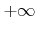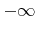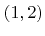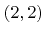Next: 4.2.2 Offset Iterator Up: 4.2 Sequential Data Access Previous: 4.2 Sequential Data Access

## 4.2.1 Basic Iterator

The basic iterator traverses the H-RLE data structure in sequential order and stops at every segment, thus at every defined grid point and at every undefined run. The iterator can be moved either forward or backward. Each step requires constant time on average. Since the number of segments is proportional to the number of defined grid points (see Section 3.5.4), a traversal of the entire data structure is possible in linear time.

The iterator allows access to all stored information of the current segment:

• The iterator provides a function that returns the minimum and maximum index vectors of the current segment. If the segment contains only a single grid point, as is the case for defined grid points, both index vectors are identical.
• The LS sign can be queried for the current segment. This is also possible for segments which are undefined runs. In this case the sign can be retrieved from the run code.
• If the current segment is a defined grid point, its LS value can be accessed using the iterator. For undefined runsoris returned instead. Floating-point data types fulfilling the IEEE standard for binary floating-point arithmetic  provide numerical representations forand. Alternatively, the maximum and minimum representable value can be used instead.

Figure 4.3b shows a two-dimensional example of a H-RLE data structure with sequentially numbered segments. The numbering corresponds to the traversal sequence of the iterator. The second position refers to the positive undefined run which contains the grid points,, and. Hence, in this case the minimum and maximum index vectors areand, respectively.

Aside from stepping forward and backward the basic iterator can also be moved to any segment, specified by an index vector belonging to that segment. However, this corresponds to a random access operation with a logarithmic complexity in the worst case.Next: 4.2.2 Offset Iterator Up: 4.2 Sequential Data Access Previous: 4.2 Sequential Data Access

Otmar Ertl: Numerical Methods for Topography Simulation